#News, Views & Insights

Browse by Topic
Related Topics

In the previous post in this series, we looked at how to model a stabilized inverted pendulum using the control systems design features in Mathematica 8. We were quickly able to simulate a linearly controlled cart-and-pendulum system, and show that it is stable against some fairly large perturbations.

But what about a double (or triple or quadruple… ) pendulum? A general n-link pendulum is depicted below. In this post we’ll see how to derive the equations of motions for this system, find out whether we can stabilize it with a linear control scheme, and produce some animations of the results.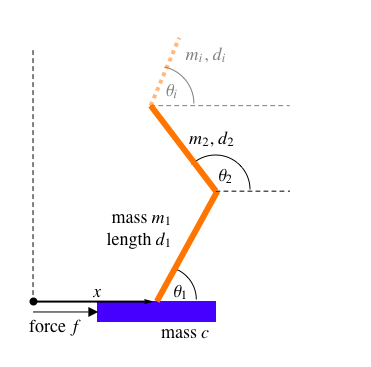For the single pendulum case from the previous post we derived the equations of motion manually, but for the n-link case it is much easier to derive them programmatically. I’ll show one way to do this step by step, using a Lagrangian mechanics formulation. If you prefer, you could easily skip ahead from here to the control theory portion of the post.

The n + 1 generalized coordinates describing the n-link pendulum depicted above are the cart position x(t) and the angles of each arm θi(t):The n + 1 generalized velocities are the time derivatives of the coordinates: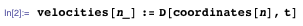Together these comprise the dynamical variables of the n-link pendulum. For the double (“2-link”) pendulum, for example, the dynamical variables are: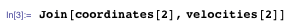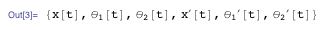The n + 1 generalized external forces are just the control force f(t) acting on the cart and zero forces acting on each of the other n generalized coordinates: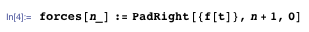For example, the generalized external forces acting on the triple pendulum are: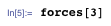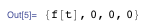Now we need to compute the Lagrangian of the n-link pendulum. It helps to define a couple of other properties of the system first, starting with the location of the hinge of each of the n arms. The hinge of the first arm is at the horizontal position of the cart, with a height of zero: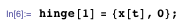The location of the hinge of each other arm depends on the location of the previous hinge in the following simple way: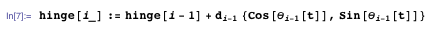For example, the hinge of the second arm is at the end of the first arm: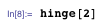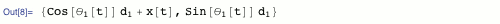We assume the arms have uniform linear density, so the center of mass of the ith arm is halfway along it: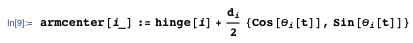The kinetic energy of the ith arm is 1/2 mi v.v, where v is the velocity of its center of mass: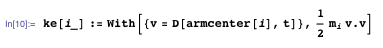In our dimensionless units in which the gravitational acceleration g = 1, the potential energy of the ith arm is its mass multiplied by the height of its center of mass: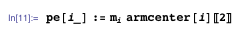(The 〚2〛 gives the second component of armcenter[i], which is the height.)

The Lagrangian of the cart is just its kinetic energy, cx′(t)2⁄2, so the overall Lagrangian of the n-link pendulum is: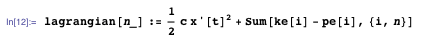Now we are ready to apply the Euler–Lagrange equations and get the equations of motion: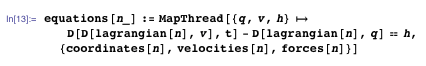(The MapThread function gives the Euler–Lagrange equation,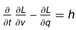, for each corresponding generalized coordinate q, generalized velocity v, and generalized force h.)

OK! After a bit of work, we have the equations of motion for any n-link pendulum. Let’s take a look at a few of them.

For n = 0 (a cart without any pendulum), we just get Newton’s second law relating the cart’s mass c and acceleration x″(t) to the control force f(t):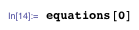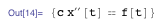So far, so good. For n = 1 we get the same equations of motion as we used in the first post in this series: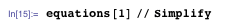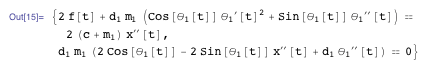For n = 2, a cart-and-double-pendulum system, the explicit equations of motion are fairly complicated: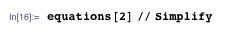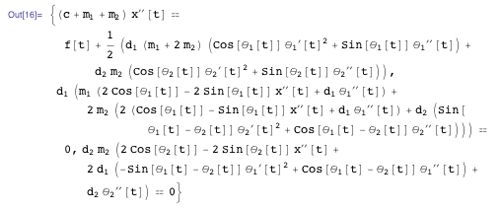The equations become much longer for larger n, but we will happily use them without ever looking at them.

(If you do want to see the equations of motion for, say, a quadruple pendulum printed out in full, just download the Computable Document Format (CDF) file for this post and evaluate equations. The output is about five pages long on-screen, which is exactly why you don’t want to derive these things by hand.)

We used symbolic math and programming to set up the equations of motion. Now we can immediately use Mathematica‘s numerics and control systems features to simulate and stabilize them. This is what’s great about having all these areas integrated into one system!

We’ll investigate the concrete case c = mi = di = 1: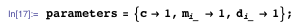(The mi_ means “mi for all values of i”.)

It’s handy to define a function initial[n] that generates some slightly non-upright initial conditions at t = 0 for an n-link pendulum. For example, here are slightly non-upright initial conditions for a single pendulum: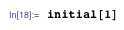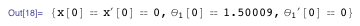Note that the pendulum is exactly upright when each θi = π ⁄ 2 ≈ 1.5708. (Download the CDF file to see the definition of initial[n], or to substitute your own.)

We’ll start by simulating a double pendulum with no control force, f(t) = 0, corresponding to making no attempt to balance the pendulum: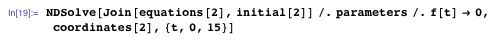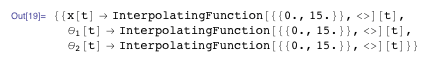Here’s an animation of the solution: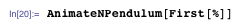(As in the previous post, you can see the definition of the function AnimateNPendulum by downloading the CDF file.)

Once again, it’s convenient to combine the above steps into a single function: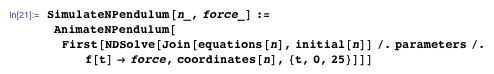Using this function, here’s a triple pendulum falling over, with no attempt to control it: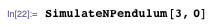It gets messy fast, but we’ll be able to steady it!

First, we need a state space model of the n-link pendulum, linearized around the equilibrium point. The function StateSpaceModel automatically generates this for us from our nonlinear equations of motion: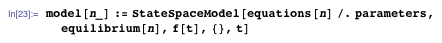The function equilibrium[n] just gives the unstable (upright) equilibrium point for an n-link pendulum: θi = π ⁄ 2; everything else = 0. For example, for a double pendulum: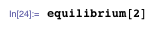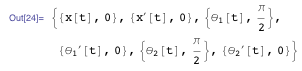Here is the linearized state space model for our double pendulum: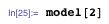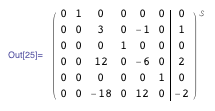As we discussed in the previous post, each column in this augmented matrix describes the immediate effect of one of the dynamical variables (or the control force) on each of the other dynamical variables.

We can now compute a linear control force for this system. For a double pendulum, for example, the linear control force will have the form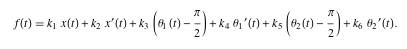LQRegulatorGains gives values for the feedback gains ki: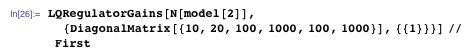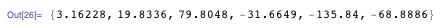As discussed in the previous post, the second argument to LQRegulatorGains specifies a quadratic cost function whose integral over time is minimized (in the linearized model). The exact cost function specified above is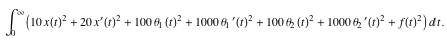The coefficients in the quadratic cost function may represent actual costs of deviations of the system from the desired equilibrium state, or they may be chosen according to other criteria, such as robustness against certain types of perturbations. The cost coefficients I’ve used here are a guess, based on which dynamical variables I suspect are the most important for keeping the pendulum from falling over.

Here is a generalization of my guessed cost coefficients to an n-link pendulum: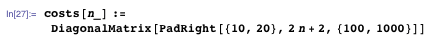The corresponding feedback gains are: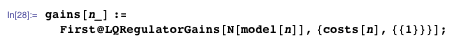And the control force is: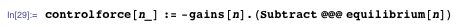Our control force for a double pendulum is: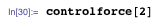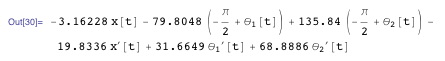How well does it work?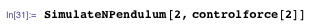Very graceful! Here’s the stabilized triple pendulum (compare it to the freely falling triple pendulum above):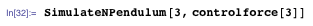Some pretty nifty maneuvering is needed to catch the quadruple pendulum before it falls over: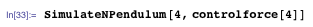Let’s see what sort of bumping around the double pendulum can handle. We’ll apply generalized forces h1(t) and h2(t) to the coordinates θ1(t) and θ2(t) respectively. (We are effectively applying torques to the first and second hinges of the pendulum.)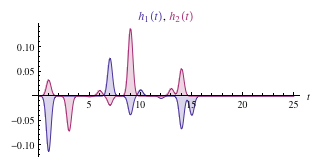(Download the CDF file to see the definitions of h1(t) and h2(t), or to substitute your own.)

Here’s the bumped double pendulum:

It’s also interesting to directly plot the generalized coordinates x(t), θ1(t), and θ2(t), and compare with the forces h1(t) and h2(t) and the control force f(t):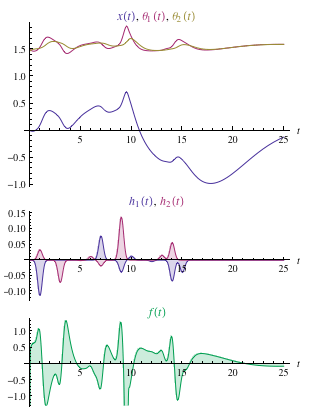With sufficiently large perturbations, linear control systems such as the ones we have been exploring will fail to recover (and will spiral out of control). Moreover, for n-link pendulums, larger values of n require smaller angular perturbations to knock them over, and in real life there are always perturbations (sound, heat, wind… ). In practice it’s hard to build physical demonstrations of n-link pendulums with more than about three or four links.

Nevertheless, it’s very interesting to explore these idealized systems. There are lots of things you can try with the downloadable CDF file for this post. For example, with different choices for the cost function, you can make linear control systems for inverted n-link pendulums that can recover from different (including larger) initial perturbations. Or, with different lengths and masses of the pendulum arms (and no control force), you can explore the dynamics of n-link pendulums, which are very interesting by themselves. Try it out!

In the next post in this series, we’ll look at some more generalizations and variations on this problem, including inverted pendulums in 3D.